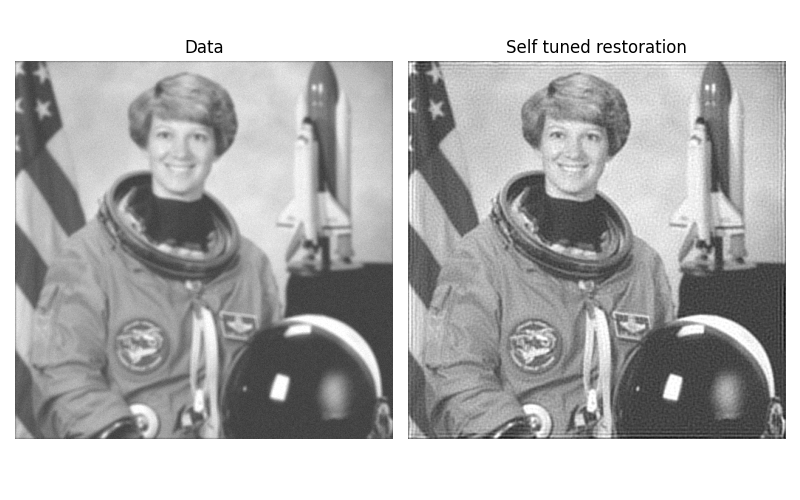# Image Deconvolution¶

In this example, we deconvolve a noisy version of an image using Wiener and unsupervised Wiener algorithms. These algorithms are based on linear models that can’t restore sharp edge as much as non-linear methods (like TV restoration) but are much faster.

## Wiener filter¶

The inverse filter based on the PSF (Point Spread Function), the prior regularization (penalisation of high frequency) and the tradeoff between the data and prior adequacy. The regularization parameter must be hand tuned.

## Unsupervised Wiener¶

This algorithm has a self-tuned regularization parameters based on data learning. This is not common and based on the following publication . The algorithm is based on an iterative Gibbs sampler that draw alternatively samples of posterior conditional law of the image, the noise power and the image frequency power.```import numpy as np
import matplotlib.pyplot as plt

from skimage import color, data, restoration

rng = np.random.default_rng()

astro = color.rgb2gray(data.astronaut())
from scipy.signal import convolve2d as conv2
psf = np.ones((5, 5)) / 25
astro = conv2(astro, psf, 'same')
astro += 0.1 * astro.std() * rng.standard_normal(astro.shape)

deconvolved, _ = restoration.unsupervised_wiener(astro, psf)

fig, ax = plt.subplots(nrows=1, ncols=2, figsize=(8, 5),
sharex=True, sharey=True)

plt.gray()

ax.imshow(astro, vmin=deconvolved.min(), vmax=deconvolved.max())
ax.axis('off')
ax.set_title('Data')

ax.imshow(deconvolved)
ax.axis('off')
ax.set_title('Self tuned restoration')

fig.tight_layout()

plt.show()
```

Total running time of the script: ( 0 minutes 0.746 seconds)

Gallery generated by Sphinx-Gallery